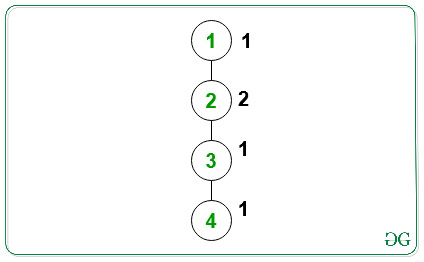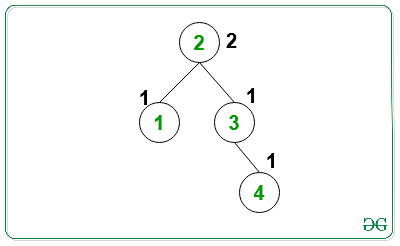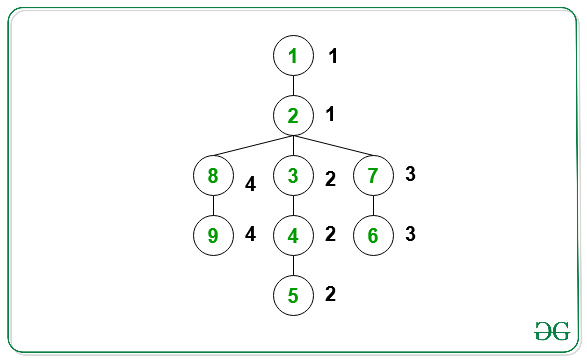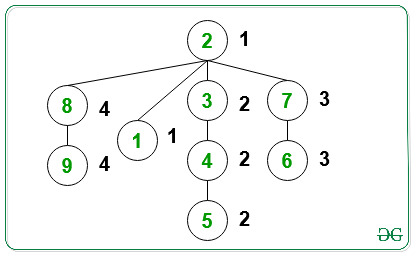# Find a node such that all paths from that node to leaf nodes are of the same color

• Difficulty Level : Hard
• Last Updated : 18 Jun, 2021

Given a 2D array edges[][] of type { X, Y } representing that there is an edge between the node X and Y in a tree, and an array color[] representing the value of the color of the ith node, the task is to find a root node of the tree such that all the child nodes of the root node on the same path have the same value of the color. If multiple solutions exist, then print any one of them. Otherwise, print -1.

Examples:

Attention reader! Don’t stop learning now. Get hold of all the important DSA concepts with the DSA Self Paced Course at a student-friendly price and become industry ready.  To complete your preparation from learning a language to DS Algo and many more,  please refer Complete Interview Preparation Course.

In case you wish to attend live classes with experts, please refer DSA Live Classes for Working Professionals and Competitive Programming Live for Students.

Input:Output:
Explanation:All the child nodes on the path from the root node(= 2) to the leaf node(= 1) have the same value of the color(= 1).
All the child nodes on the path from the root node(= 2) to the leaf node(= 4) have the same value of the color(= 1).
Therefore, the required output is 2.

Input:Output:
Explanation:All the child nodes on the path from the root node(=2) to the leaf node(=9) have the same value of the color(= 4).
All the child nodes on the path from the root node(=2) to the leaf node(=1) have the same value of the color(= 1).
All the child nodes on the path from the root node(=2) to the leaf node(=5) have the same value of the color(= 2).
All the child nodes on the path from the root node(=2) to the leaf node(=6) have the same value of the color(= 3).

Approach: The idea is to iterate over all possible nodes of the tree. For every ith node, check if it satisfies the condition of the root node or not using DFS. If found to be true, then print the node. Otherwise, print -1. Follow the steps below to solve the problem:

• Initialize a variable, say root, to store the root node of the tree that satisfies the condition.
• Iterate over all possible nodes of the tree. Consider every ith node of the tree as root node and check if all the child nodes on the path from root node to the leaf node have the same color or not using DFS. If found to be true, then print the node.
• Otherwise, print -1.

Below is the implementation of the above approach:

## C++

 `// C++ program to implement``// the above approach` `#include ``using` `namespace` `std;` `// Function to perform dfs on the tree``bool` `dfs(``int` `node, ``int` `c, vector<``int``> adj[],``         ``int` `color[], ``int` `visited[])``{` `    ``// Mark visited node as true``    ``visited[node] = ``true``;` `    ``// If color does not match with``    ``// previous node on the same path``    ``if` `(color[node] != c) {``        ``return` `false``;``    ``}` `    ``// Check if current subtree``    ``// has all same colored nodes``    ``int` `f = 1;` `    ``// Traverse all unvisited neighbors``    ``// node of the tree``    ``for` `(``int` `j = 0; j < adj[node].size(); j++) {` `        ``// Stores neighbors node``        ``// of the tree``        ``int` `neighbor = adj[node][j];` `        ``// If current node is not``        ``// already visited``        ``if` `(!visited[neighbor]) {` `            ``if` `(dfs(neighbor, c, adj,``                    ``color, visited)``                ``== ``false``) {` `                ``// Update f``                ``f = 0;``                ``break``;``            ``}``        ``}``    ``}``    ``return` `f;``}` `// Function to find the root node of``// the tree such that all child nodes``// on the same path have the same color``void` `findNode(``int` `edges[],``              ``int` `color[], ``int` `n)``{` `    ``// Store the adjacency list``    ``vector<``int``> adj[n + 1];` `    ``// Traverse all edges and form``    ``// the adjacency list``    ``for` `(``int` `i = 0; i < n - 1; i++) {``        ``int` `a = edges[i];``        ``int` `b = edges[i];``        ``adj[a].push_back(b);``        ``adj[b].push_back(a);``    ``}` `    ``// Store the root node such that all``    ``// child nodes on the same path have``    ``// the same color``    ``int` `ans = -1;` `    ``// Iterate over all possible``    ``// nodes of the tree``    ``for` `(``int` `i = 1; i <= n; i++) {` `        ``// Check if node i satisfies``        ``// the condition of root node``        ``int` `f = 1;` `        ``// Check if a node has been``        ``// visited or not``        ``int` `visited[n + 1] = { ``false` `};` `        ``// Mark visited[i] as true``        ``visited[i] = ``true``;` `        ``// Traverse all the neighbors``        ``// of node i``        ``for` `(``int` `j = 0; j < adj[i].size(); j++) {` `            ``// Stores the current neighbor``            ``int` `neighbor = adj[i][j];` `            ``// Perform DFS for current neighbor``            ``if` `(dfs(neighbor, color[neighbor],``                    ``adj, color, visited)``                ``== ``false``) {` `                ``// Update f``                ``f = 0;``                ``break``;``            ``}``        ``}` `        ``if` `(f == 1) {``            ``ans = i;``            ``break``;``        ``}``    ``}` `    ``// Print the answer``    ``cout << ans;``}` `// Driver Code``int` `main()``{` `    ``int` `n = 9;``    ``int` `color[n + 1] = { -1, 1, 1, 2, 2,``                         ``2, 3, 3, 4, 4 };` `    ``int` `edges[] = { { 1, 2 }, { 2, 3 },``                       ``{ 3, 4 }, { 4, 5 },``                       ``{ 2, 7 }, { 7, 6 },``                       ``{ 2, 8 }, { 8, 9 } };` `    ``findNode(edges, color, n);` `    ``return` `0;``}`

## Java

 `// Java program to implement``// the above approach``import` `java.util.*;``class` `GFG``{` `// Function to perform dfs on the tree``static` `boolean` `dfs(``int` `node, ``int` `c, Vector adj[],``         ``int` `color[], ``boolean` `visited[])``{` `    ``// Mark visited node as true``    ``visited[node] = ``true``;` `    ``// If color does not match with``    ``// previous node on the same path``    ``if` `(color[node] != c)``    ``{``        ``return` `false``;``    ``}` `    ``// Check if current subtree``    ``// has all same colored nodes``    ``boolean` `f = ``true``;` `    ``// Traverse all unvisited neighbors``    ``// node of the tree``    ``for` `(``int` `j = ``0``; j < adj[node].size(); j++)``    ``{` `        ``// Stores neighbors node``        ``// of the tree``        ``int` `neighbor = adj[node].get(j);` `        ``// If current node is not``        ``// already visited``        ``if` `(!visited[neighbor])``        ``{` `            ``if` `(dfs(neighbor, c, adj,``                    ``color, visited) == ``false``)``            ``{` `                ``// Update f``                ``f = ``false``;``                ``break``;``            ``}``        ``}``    ``}``    ``return` `f;``}` `// Function to find the root node of``// the tree such that all child nodes``// on the same path have the same color``static` `void` `findNode(``int` `edges[][],``              ``int` `color[], ``int` `n)``{` `    ``// Store the adjacency list``    ``Vector []adj = ``new` `Vector[n + ``1``];``    ``for``(``int` `i = ``0``; i < n + ``1``; i++)``        ``adj[i] = ``new` `Vector();` `    ``// Traverse all edges and form``    ``// the adjacency list``    ``for` `(``int` `i = ``0``; i < n - ``1``; i++)``    ``{``        ``int` `a = edges[i][``0``];``        ``int` `b = edges[i][``1``];``        ``adj[a].add(b);``        ``adj[b].add(a);``    ``}` `    ``// Store the root node such that all``    ``// child nodes on the same path have``    ``// the same color``    ``int` `ans = -``1``;` `    ``// Iterate over all possible``    ``// nodes of the tree``    ``for` `(``int` `i = ``1``; i <= n; i++)``    ``{` `        ``// Check if node i satisfies``        ``// the condition of root node``        ``int` `f = ``1``;` `        ``// Check if a node has been``        ``// visited or not``        ``boolean` `[]visited = ``new` `boolean``[n + ``1``];` `        ``// Mark visited[i] as true``        ``visited[i] = ``true``;` `        ``// Traverse all the neighbors``        ``// of node i``        ``for` `(``int` `j = ``0``; j < adj[i].size(); j++)``        ``{` `            ``// Stores the current neighbor``            ``int` `neighbor = adj[i].get(j);` `            ``// Perform DFS for current neighbor``            ``if` `(dfs(neighbor, color[neighbor],``                    ``adj, color, visited) == ``false``)``            ``{` `                ``// Update f``                ``f = ``0``;``                ``break``;``            ``}``        ``}``        ``if` `(f == ``1``)``        ``{``            ``ans = i;``            ``break``;``        ``}``    ``}` `    ``// Print the answer``    ``System.out.print(ans);``}` `// Driver Code``public` `static` `void` `main(String[] args)``{` `    ``int` `n = ``9``;``    ``int` `color[] = { -``1``, ``1``, ``1``, ``2``, ``2``,``                         ``2``, ``3``, ``3``, ``4``, ``4` `};``    ``int` `edges[][] = { { ``1``, ``2` `}, { ``2``, ``3` `},``                       ``{ ``3``, ``4` `}, { ``4``, ``5` `},``                       ``{ ``2``, ``7` `}, { ``7``, ``6` `},``                       ``{ ``2``, ``8` `}, { ``8``, ``9` `} };``    ``findNode(edges, color, n);``}``}` `// This code is contributed by 29AjayKumar`

## Python3

 `# Python program to implement``# the above approach``from` `typing ``import` `List` `# Function to perform dfs on the tree``def` `dfs(node: ``int``, c: ``int``, adj: ``List``[``List``[``int``]],``        ``color: ``List``[``int``],``        ``visited: ``List``[``int``]) ``-``> ``bool``:` `    ``# Mark visited node as true``    ``visited[node] ``=` `True` `    ``# If color does not match with``    ``# previous node on the same path``    ``if` `(color[node] !``=` `c):``        ``return` `False` `    ``# Check if current subtree``    ``# has all same colored nodes``    ``f ``=` `1` `    ``# Traverse all unvisited neighbors``    ``# node of the tree``    ``for` `j ``in` `range``(``len``(adj[node])):` `        ``# Stores neighbors node``        ``# of the tree``        ``neighbor ``=` `adj[node][j]` `        ``# If current node is not``        ``# already visited``        ``if` `(``not` `visited[neighbor]):``            ``if` `not` `dfs(neighbor, c, adj, color, visited):` `                ``# Update f``                ``f ``=` `0``                ``break``    ``return` `f` `# Function to find the root node of``# the tree such that all child nodes``# on the same path have the same color``def` `findNode(edges: ``List``[``List``[``int``]], color: ``List``[``int``], n: ``int``) ``-``> ``None``:` `    ``# Store the adjacency list``    ``adj ``=` `[[] ``for` `_ ``in` `range``(n ``+` `1``)]` `    ``# Traverse all edges and form``    ``# the adjacency list``    ``for` `i ``in` `range``(n ``-` `1``):``        ``a ``=` `edges[i][``0``]``        ``b ``=` `edges[i][``1``]``        ``adj[a].append(b)``        ``adj[b].append(a)` `    ``# Store the root node such that all``    ``# child nodes on the same path have``    ``# the same color``    ``ans ``=` `-``1` `    ``# Iterate over all possible``    ``# nodes of the tree``    ``for` `i ``in` `range``(``1``, n ``+` `1``):` `        ``# Check if node i satisfies``        ``# the condition of root node``        ``f ``=` `1` `        ``# Check if a node has been``        ``# visited or not``        ``visited ``=` `[``False` `for` `_ ``in` `range``(n ``+` `1``)]` `        ``# Mark visited[i] as true``        ``visited[i] ``=` `True` `        ``# Traverse all the neighbors``        ``# of node i``        ``for` `j ``in` `range``(``len``(adj[i])):` `            ``# Stores the current neighbor``            ``neighbor ``=` `adj[i][j]` `            ``# Perform DFS for current neighbor``            ``if` `not` `dfs(neighbor, color[neighbor],``                       ``adj, color, visited):` `                ``# Update f``                ``f ``=` `0``                ``break` `        ``if` `(f ``=``=` `1``):``            ``ans ``=` `i``            ``break` `    ``# Print the answer``    ``print``(ans)` `# Driver Code``if` `__name__ ``=``=` `"__main__"``:` `    ``n ``=` `9``    ``color ``=` `[``-``1``, ``1``, ``1``, ``2``, ``2``, ``2``, ``3``, ``3``, ``4``, ``4``]``    ``edges ``=` `[[``1``, ``2``], [``2``, ``3``], [``3``, ``4``], [``4``, ``5``], [``2``, ``7``], [``7``, ``6``], [``2``, ``8``], [``8``, ``9``]]``    ``findNode(edges, color, n)` `# This code is contributed by sanjeev2552`

## C#

 `// C# program to implement``// the above approach``using` `System;``using` `System.Collections.Generic;``class` `GFG``{` `// Function to perform dfs on the tree``static` `bool` `dfs(``int` `node, ``int` `c, List<``int``> []adj,``         ``int` `[]color, ``bool` `[]visited)``{` `    ``// Mark visited node as true``    ``visited[node] = ``true``;` `    ``// If color does not match with``    ``// previous node on the same path``    ``if` `(color[node] != c)``    ``{``        ``return` `false``;``    ``}` `    ``// Check if current subtree``    ``// has all same colored nodes``    ``bool` `f = ``true``;` `    ``// Traverse all unvisited neighbors``    ``// node of the tree``    ``for` `(``int` `j = 0; j < adj[node].Count; j++)``    ``{` `        ``// Stores neighbors node``        ``// of the tree``        ``int` `neighbor = adj[node][j];` `        ``// If current node is not``        ``// already visited``        ``if` `(!visited[neighbor])``        ``{` `            ``if` `(dfs(neighbor, c, adj,``                    ``color, visited) == ``false``)``            ``{` `                ``// Update f``                ``f = ``false``;``                ``break``;``            ``}``        ``}``    ``}``    ``return` `f;``}` `// Function to find the root node of``// the tree such that all child nodes``// on the same path have the same color``static` `void` `findNode(``int` `[,]edges,``              ``int` `[]color, ``int` `n)``{` `    ``// Store the adjacency list``    ``List<``int``> []adj = ``new` `List<``int``>[n + 1];``    ``for``(``int` `i = 0; i < n + 1; i++)``        ``adj[i] = ``new` `List<``int``>();` `    ``// Traverse all edges and form``    ``// the adjacency list``    ``for` `(``int` `i = 0; i < n - 1; i++)``    ``{``        ``int` `a = edges[i, 0];``        ``int` `b = edges[i, 1];``        ``adj[a].Add(b);``        ``adj[b].Add(a);``    ``}` `    ``// Store the root node such that all``    ``// child nodes on the same path have``    ``// the same color``    ``int` `ans = -1;` `    ``// Iterate over all possible``    ``// nodes of the tree``    ``for` `(``int` `i = 1; i <= n; i++)``    ``{` `        ``// Check if node i satisfies``        ``// the condition of root node``        ``int` `f = 1;` `        ``// Check if a node has been``        ``// visited or not``        ``bool` `[]visited = ``new` `bool``[n + 1];` `        ``// Mark visited[i] as true``        ``visited[i] = ``true``;` `        ``// Traverse all the neighbors``        ``// of node i``        ``for` `(``int` `j = 0; j < adj[i].Count; j++)``        ``{` `            ``// Stores the current neighbor``            ``int` `neighbor = adj[i][j];` `            ``// Perform DFS for current neighbor``            ``if` `(dfs(neighbor, color[neighbor],``                    ``adj, color, visited) == ``false``)``            ``{` `                ``// Update f``                ``f = 0;``                ``break``;``            ``}``        ``}``        ``if` `(f == 1)``        ``{``            ``ans = i;``            ``break``;``        ``}``    ``}` `    ``// Print the answer``    ``Console.Write(ans);``}` `// Driver Code``public` `static` `void` `Main(String[] args)``{``    ``int` `n = 9;``    ``int` `[]color = { -1, 1, 1, 2, 2,``                         ``2, 3, 3, 4, 4 };``    ``int` `[,]edges = { { 1, 2 }, { 2, 3 },``                       ``{ 3, 4 }, { 4, 5 },``                       ``{ 2, 7 }, { 7, 6 },``                       ``{ 2, 8 }, { 8, 9 } };``    ``findNode(edges, color, n);``}``}` `// This code is contributed by 29AjayKumar`

## Javascript

 ``
Output:
`2`

Time complexity: O(N2)
Auxiliary space: O(N)

My Personal Notes arrow_drop_up